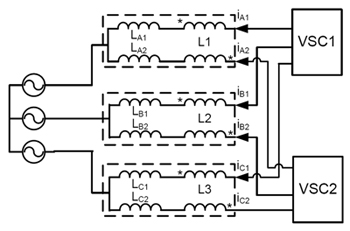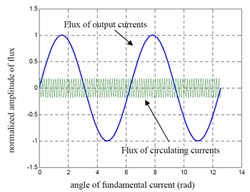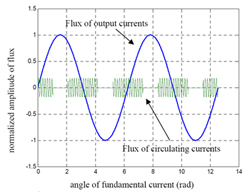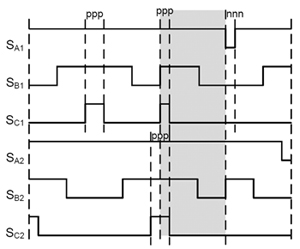LIBRARY

# Total Flux Minimization Control for Integrated Inter-Phase Inductors in Paralleled, Interleaved Three-Phase Two-Level Voltage-Source Converters With Discontinuous Space-vector Modulation

Year: 2013Fig. 1. Interleaved three phase rectifier.
Three-phase voltage source converters (VSCs) are very popular in AC medium and high power applications. Paralleled three-phase VSCs have the benefits of higher power, modularity, redundancy and, flexibility. Interleaving can reduce output harmonic currents in a paralleled VSCs system, which has the potential to increase power density; a desired effect for many applications such as vehicle and aircraft. This benefit is more significant for rectifier applications as shown in Fig.1, where the interphase inductor that is used to suppress the circulating current generated by interleaving can be integrated with the boost inductors which already exist in the system.

Due to the phase-shifting of the two converters in the interleaving topology, the total flux in the integrated input inductors will include the low frequency part that is related to the output current, and the high frequency part that is related to the circulating current, as shown in Fig. 2. Without proper control, those two parts will add together and the total flux will be larger than the non-interleaving case. However, with proper control of the circulating current, the high frequency flux can be controlled as in Fig.2b, then the total flux is minimized and the input inductor size can be smaller.

In the interleaving topology shown as Fig.1, when a discontinuous PWM modulation method is used, the circulating current will not change after the switches are clamped in one phase. Total flux can be minimized if the circulating current in this phase can be controlled to zero before switches are clamped. The amplitude of the CM circulating current can be adjusted by controlling the portion between ppp and nnn in the zero vector duration as shown in Fig.3. Since the CM circulating current is part of the total circulating current, the amplitude of the total circulating current can also be adjusted to zero by controlling the amplitude of the CM circulating current. Using this method, the total flux of the input inductor can be minimized and the inductor size can be reduced.Fig. 2 (a) Flux without control.Fig. 2. (b) Flux with flux minimization control.Fig. 3. Control method for flux minimization control.